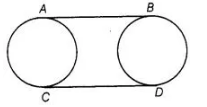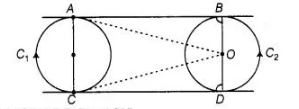# in figure, AB and CD are common tangents

Question:

in figure, AB and CD are common tangents to two circles of equal radii. Prove that AB = CD.Solution:

Given AB and CD are tangents to two circles of equal radii.

To prove                                               AB = CDConstruction Join $O A, O C, O^{\prime} B$ and $O^{\prime} D$

Proof Now, $\angle O A B=90^{\circ}$

[tangent at any point of a circle is perpendicular to radius through the point of contact]

Thus, $A C$ is a straight line.

Also, $\angle O A B+\angle O C D=180^{\circ}$

$\therefore \quad A B \| C D$

Similarly, $B D$ is a straight line

and $\angle O^{\prime} B A=\angle O^{\prime} D C=90^{\circ}$

Also, $A C=B D$ [radii of two circles are equal]

In quadrilaterai $A B C D$, $\angle A=\angle B=\angle C=\angle D=90^{\circ}$

and $A C=B D$

$A B C D$ is a rectangle

Hence, $A B=C D$ [opposite sides of rectangle are equal]